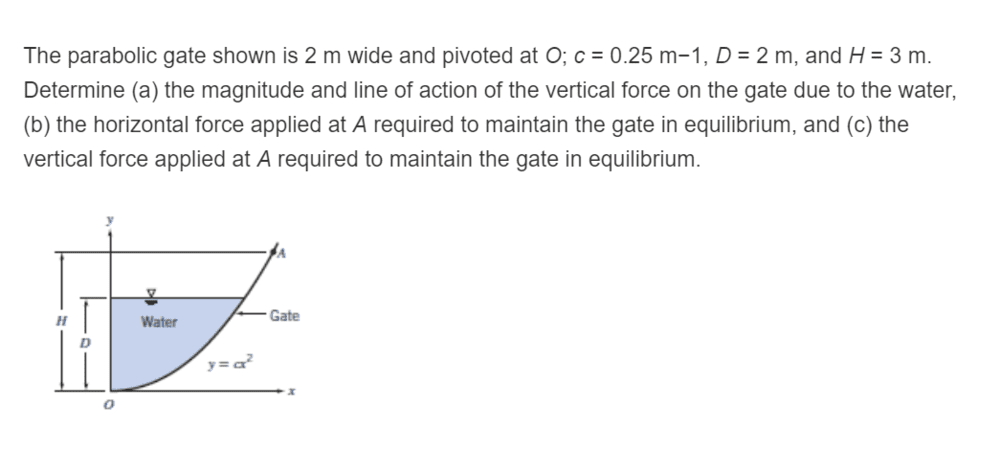# Fluid Mechanics Problem: Water Forces on a Parabolic Gate

• Engineering
ktjj4
Homework Statement:
Hello, I'm a little confused for this part of the fluid mechanics problem. I got through calculating the forces of the water on the gate but then it asked me to calculate the horizontal and vertical forces at a certain point (A) on the curved gate to keep equilibrium. I know I have to take the moment about the origin (O) but I am unsure as to why I only have to consider one of the component forces (F_Ax) but not the other (F_Ay). I'm not sure if this is a conceptual thing or if my knowledge of moments is missing something.
Relevant Equations:
Sum of M = -Fv(x') + Fh(D-y') - F_Ax(H)Last edited by a moderator: